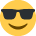# Task 1.6 - Assessment 1 and 2 (B and C)

Happy Sunday!

I have my budgeting exam in less than 2 weeks and I am currently going through the online assessments. I'm happy with all tasks except 1.6. and C).. I've looked at the answers and still cannot work out how they got them. Would anyone be able to help at all? I'm really struggling and sent days trying to work it out but haven't gotten anywhere! Thank you so much in advance.

• Sample assessment one Task 1.6 (b) workings:-
Flexed Standard cost 6000 units x 1.6 kg x £14.50 (per kg) = 139,200
Actual material price per kg = £142560 / 9900 kg = 14.4
Actual material used per item = 9900kgs / 6000 units = 1.65kg
Price variance = actual quantity at actual price minus actual quantity at budget (standard) price = 142560 - (9900 kg x £14.50) = 143,550 = 990 Favourable (the standard price is higher than the actual)
Usage variance = actual usage at std price minus standard usage at std price (9900 x £14.50) = 143550 - (6000 x 1.6 kg x £14.50) = 139200 = 4350 Adverse

Total Cost variance = The difference between the standard (budgeted) cost of 6000 units and the actual cost. 139200 - 142560 = 3360 (this is also the total of the other two variances)
Cost variance percentage = variance / budget (standard) = 3360/139200 x100 = 2.4%

First you need to flex the labour budget to the correct number of items made as follows:-
Budgeted time for one item = 0.2 hour - budgeted hours for actual production = 18800 x 0.2 =3760 x budgeted rate = £18 (divide the budgeted cost with the budgeted hours:- 65880 /3660)
Therefore standard labour cost of production = 67680
Labour rate variance =
Actual hours x actual rate - actual hours x budget rate = 82720 - (4700 hours x £18 per hour) 84600 = 1880 Favourable
Labour efficiency variance =
Actual hours at budget rate - standard hours at budget rate = 84600 - (3760 x £18) = 67680 Adverse

Remember that budget means standard - I have interchanged these words.

I have only included the solution for the assessment number one, if you need any more help please let me know.
• Thank you so much Sunflower1o2. I understand what you have done, I just have to remember and learn it !I really appreciate your help and effort with this.
• That's a great help Sunflower102 i was stuck on the same question - Alexbraam did you pass? i have mine in 2weeks....